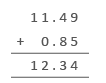Practice Unlimited Questions

Step 1: Align the decimal points.

See working examples below.

#### 1. Bob has 0.3 kg of butter and Bill has 0.4 kg of butter. How much butter do the two boys have together?

Add 0.3 and 0.4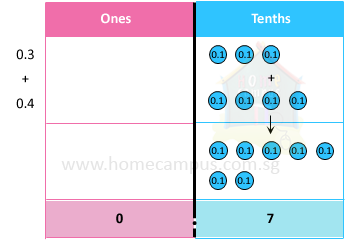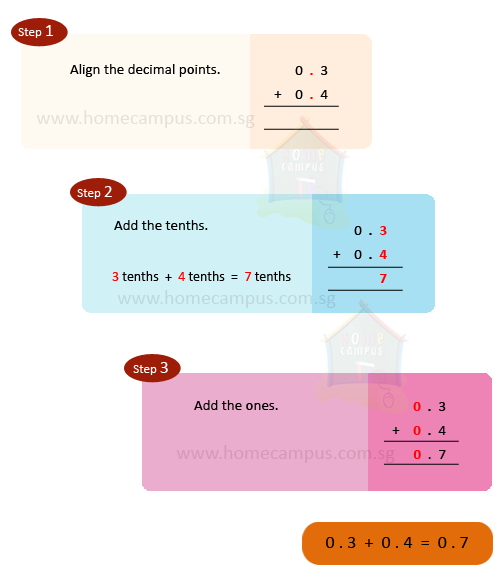The two boys have 0.7 kg of butter altogether.

#### 2. Bob has 0.7 L of milk and Bill has 0.9 L of milk. How much milk do the two boys have together?

Add 0.7 and 0.9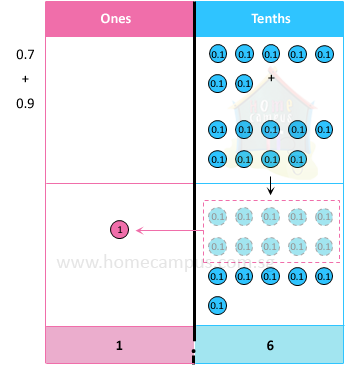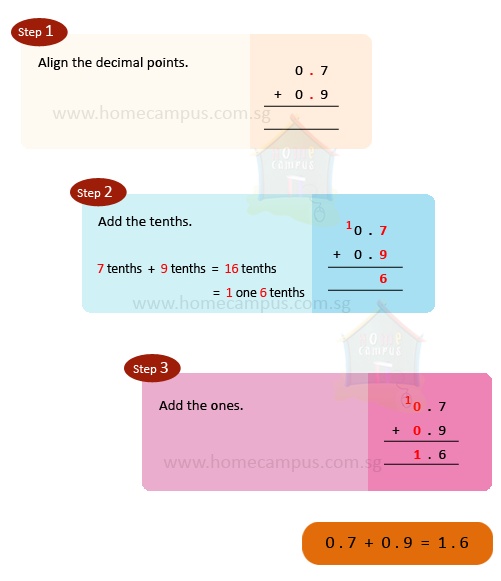The two boys have 1.6 L of milk together.

#### 3. Bob has 3.67 kg of flour and Bill has 2.55 kg of flour. How much flour do the two boys have together?

Add 3.67 and 2.55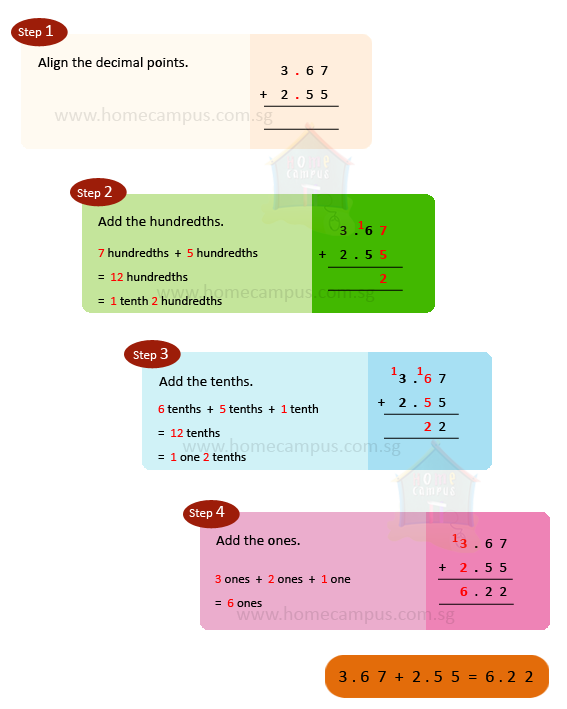The two boys have 6.22 kg of flour together.

#### 4. Serena spent \$11.49 on a diary and 85 ¢ on a pen. How much money did she spend altogether?

Cost of diary   =   \$11.49
Cost of pen   =   \$0.85
85 ¢  =  \$0.85
Total cost   =   \$11.49  +  \$0.85
=   \$12.34

She spent \$12.34 altogether.